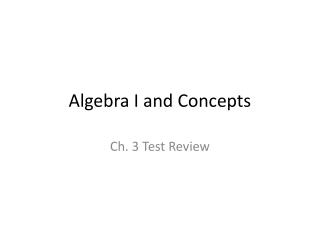DownloadDownload PresentationAlgebra I and Concepts

# Algebra I and Concepts

Télécharger la présentation## Algebra I and Concepts

- - - - - - - - - - - - - - - - - - - - - - - - - - - E N D - - - - - - - - - - - - - - - - - - - - - - - - - - -
##### Presentation Transcript

1. Algebra I and Concepts Ch. 3 Test Review

2. Directions • Get out a piece of paper, put your name and “Ch. 3 Test Review” at the top • As each slide appears, work through the problems shown. You may not finish them all, that is ok! Don’t rush, work through what you can. • When the answer slide is posted, check your answers, find and correct mistakes. Ask questions if necessary. • Finish any questions by looking up the review on my website • This will be turn in on MONDAY with your homework

3. Find the x and y intercepts of the lines graphed

4. Find the x and y intercepts of the lines graphed X-int: (-5, 0) y-int: (0, 3) X-int: (7, 0) y-int: (0, 7)

5. Find the x and y intercepts of the following equations 1) -5x + 8y = -40 2) x – 3y = 15

6. Find the x and y intercepts of the following equations 1) -5x + 8y = -40 2) x – 3y = 15 X-int: (8, 0) x-int: (15, 0) Y-int: (0, -5) y-int: (0, -5)

7. Graph using the x and y intercepts 1) 3x – 4y = 12 2) -x – 3y = 9

8. Given two functions, determine any similarities and differences of their graphs. (Hint: Use cover-up to graph) • 3x – 4y = 12 2) -x – 3y = 9

9. Find the slope of the line through the 2 points, then use the slope to classify the line as, horizontal, vertical, or oblique 1) (-3, 7) (-3, 4) 2) (-4, 3), (-2, 1) 3) (5, 2), (-6, 2)

10. Find the slope of the line through the 2 points, then use the slope to classify the line as, horizontal, vertical, or oblique 1) (-3, 7) (-3, 4) Slope: undefined Vertical Line 2) (-4, 3), (-2, 1) Slope: -1 Oblique 3) (5, 2), (-6, 2) Slope: 0 Horizontal Line

11. Word Problems! • In 2000, the cost of in-state tuition at Mizzou was \$9,000 a year. In 2013 the cost of in-state tuition is \$21,000. Find the rate of change.

12. Word Problems! • In 2000, the cost of in-state tuition at Mizzou was \$9,000 a year. In 2013 the cost of in-state tuition is \$21,000. Find the rate of change. Increasing \$923.07 per year

13. Complete the problems: The following table represents a linear function. Fill it in and identify the x and y intercepts Graph the line if the x intercept is 5, and the y-intercept is -3

14. Complete the problems: The following table represents a linear function. Fill it in and identify the x and y intercepts Graph the line if the x intercept is 5, and the y-intercept is -3 X-int: (1,0) Y-int: (0, 4)

15. Graph the following equations by filling in the table and using the points 1) y = -x + 4 3) y = 3x + 8

16. Graph the following equations by filling in the table and using the points 1) y = -x + 4 3) y = 3x + 8

17. Mrs. Huseman and Ms. Howard are going to put up Christmas lights. They lean a ladder against the house and the top of the ladder touches the house at 12 feet above the ground. The base of the ladder is 6 feet from the side of the house. • Draw a picture of the situation and label everything! • What is the slope of the ladder as a positive number?

18. Mrs. Huseman and Ms. Howard are going to put up Christmas lights. They lean a ladder against the house and the top of the ladder touches the house at 12 feet above the ground. The base of the ladder is 6 feet from the side of the house. • Draw a picture of the situation and label everything! • What is the slope of the ladder as a positive number? Slope = 2